Try out our new practice tests completely free!# Quiz 9: Modelling Volatility and CorrelationWhich of the following are problems associated with the Engle-Granger approach to modelling using cointegrated data? (I) The coefficients in the cointegrating relationship are hard to calculate (ii) This method requires the researcher to assume that one variable is the dependent variable and the others are independent variables (iii) The Engle-Granger technique can only detect one cointegrating relationship (iv) Engle-Granger does not allow the testing of hypotheses involving the actual cointegrating relationship.(Multiple Choice)

BWhich criticism of Dickey-Fuller (DF) -type tests is addressed by stationarity tests, such as the KPSS test?(Multiple Choice)

ATo induce stationarity in a deterministic trend-stationary process(Multiple Choice)

AConsider the following vector error correction (VECM) model: yt = yt-5 + 1yt-1 + 2yt-2 + 3yt-3 + 4yt-4 + ut Where yt is a k 1 vector of variables, and ut is a k 1 vector of disturbances. Which of the following statements is true of the VECM?
(Multiple Choice)What is the impact of shocks to an AR(1) with no drift if ?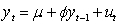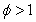(Multiple Choice)Assuming the researcher in question 19 would like to run an augmented Dickey-Fuller test instead. What is the appropriate regression she would have to run and the null hypothesis of the test?
(Multiple Choice)The plotted series in the following graph is an example of a: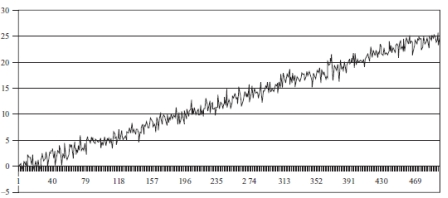(Multiple Choice)If the number of non-zero eigenvalues of the pi matrix under a Johansen test is 2, this implies that
(Multiple Choice)If a Johansen "max" test for a null hypothesis of 1 cointegrating vectors is applied to a system containing 4 variables is conducted, which eigenvalues would be used in the test?
(Multiple Choice)Which of these is a characteristic of a stationary series?
(Multiple Choice)Which one of the following best describes most series of asset prices?
(Multiple Choice)Which of the following are probably valid criticisms of the Dickey Fuller methodology? (I) The tests have a unit root under the null hypothesis and this may not be rejected due to insufficient information in the sample (ii) the tests are poor at detecting a stationary process with a unit root close to the non-stationary boundary (iii) the tests are highly complex to calculate in practice (iv) the tests have low power in small samples
(Multiple Choice)Which of the following are consequences of using non-stationary data in regressions? (I) Shocks will be persistent (II) It can lead to spurious regressions (III) t-ratios will not follow a t-distribution (IV) F-Statistic will not follow an F-distribution
(Multiple Choice)A researcher would like to test for a unit root in a series. She runs the regression . What should her null hypothesis be assuming that she adopts the Dickey-Fuller test approach?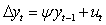(Multiple Choice)Consider the testing of hypotheses concerning the cointegrating vector(s) under the Johansen approach. Which of the following statements is correct?
(Multiple Choice)If there are three variables that are being tested for cointegration, what is the maximum number of linearly independent cointegrating relationships that there could be?
(Multiple Choice)Consider the following matrix: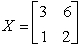What are its characteristic roots?
(Multiple Choice)What is the impact of shocks to an AR(1) with no drift if ?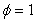(Multiple Choice)Consider the following data generating process for a series yt:Which one of the following most accurately describes the process for yt?
(Multiple Choice)You have the following data for Johansen's max rank test for cointegration between 4 international equity market indices:How many cointegrating vectors are there?
(Multiple Choice)## Filters

• Essay(0)
• Multiple Choice(0)